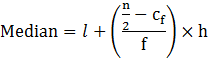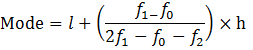Guru

# In this 100 surnames were randomly picked up from a local telephone directory and the frequency distribution of the number of letters in English alphabets in the surnames was obtained as follows: Q.6

• 0

Yesterday i was doing the question of statics chapter of exercise 14.3 of question no,6. of ncert class 10th ncert math give me the simple way for solving this question in easy way In this 100 surnames were randomly picked up from a local telephone directory and the frequency distribution of the number of letters in English alphabets in the surnames was obtained as follows:

Share

1. n = 100 &n/2 = 50

Median class = 7-10

Therefore, l = 7, Cf = 36, f = 40 & h = 3Median = 7+((50-36)/40) × 3

Median = 7+42/40

Median=8.05

Calculate the Mode:

Modal class = 7-10,

Where, l = 7, f1 = 40, f0 = 30, f2 = 16 & h = 3Mode = 7+((40-30)/(2×40-30-16)) × 3

= 7+(30/34)

= 7.88

Therefore mode = 7.88

Mean = x̄ = ∑fi xi /∑f

Mean = 825/100 = 8.25

Therefore, mean = 8.25

• 0